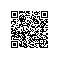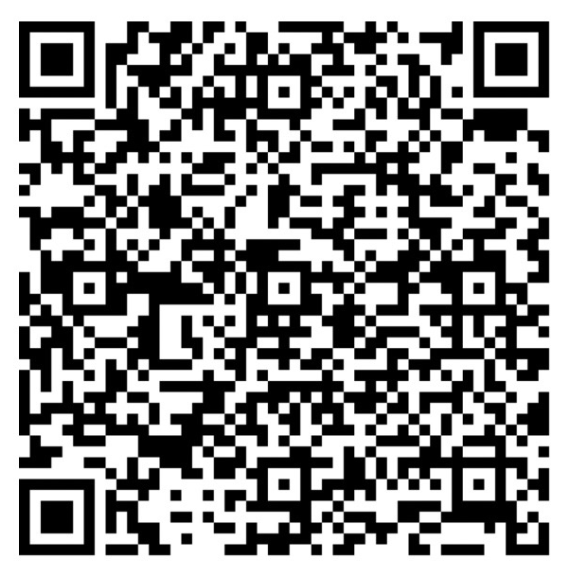# Python学习(21)--深拷贝与浅拷贝1.对象赋值

2.浅拷贝

3.深拷贝

(1)对象赋值

[python] view plain copy

1. a=4
2. b=4
3. c=4
4. print(id(4))
5. print(id(a))
6. print(id(b))
7. print(id(c))[python] view plain copy

1. a=[1,2,"a","b"]
2. b=a
3. print(b)
4. print(a)
5. print(id(a))
6. print(id(b))[python] view plain copy

1. a=[1,2,"a","b"]
2. b=a
3. a.append("c")
4. print(a)
5. print(b)
6. b.append(3)
7. print(a)
8. print(b)(2)浅拷贝

[python] view plain copy

1. import copy
2. a=[1,2,['a','b']]
3. b=copy.copy(a)
4. print(id(a))
5. print(id(b))
6. for aele in a:
7.     print(id(aele))
8. for bele in b:
9.     print(id(bele))[python] view plain copy

1. import copy
2. a=[1,2,['a','b']]
3. b=copy.copy(a)
4. b=5
5. print(a)
6. print(b)
7. print(id(a))
8. print(id(b))[python] view plain copy

1. import copy
2. a=[1,2,['a','b']]
3. b=copy.copy(a)
4. a.append("d")
5. print(a)
6. print(b)
7. print(id(a))
8. print(id(b))(3)深拷贝

[python] view plain copy

1. import copy
2. a=[1,2,['a','b']]
3. b=copy.deepcopy(a)
4. print(id(a))
5. print(id(b))
6. for aele in a:
7.     print(id(aele))
8. for bele in b:
9.     print(id(bele))[python] view plain copy

1. import copy
2. a=[1,2,['a','b']]
3. b=copy.deepcopy(a)
4. a.append('d')
5. print(a)
6. print(b)使用钉钉扫一扫加入圈子
+ 订阅